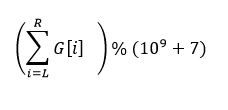# Permutations and DivisorsYouKnowWhoContest Based on SUST Int...
Limits 1s, 512 MB

Let P be a permutation of length n where each value from 1 to n occurs exactly once. A subsequence of P is called good if every element is a divisor of its immediate next element of the subsequence.

So for a permutation [2,4,1,5,3,6] of length 6, [1,3,6], , [2,6] etc are some good subsequences, where [2,3,6]—because 2 doesn't divide 3, is not. The size of a subsequence is the number of elements in it.

We define G[n] by the maximum size of a good subsequence over all permutations of length n.

Given two integers L and R, find## Input

The first line will contain an integer q(1 ≤ q ≤ 105), the number of queries you need to proceed.

Each of the next q lines contains two integers L and R(1 ≤ L ≤ R ≤ 109).

## Output

For each query output the value of the above function modulo (109+7).

## Sample

InputOutput
2
1 2
3 3

3
2uDebug

SSJ_naiM

### Statistics

87% Solution Ratio
tvirussustEarliest, Dec '19